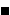# Easy Construction of Bicentric Quadrilateral II: What Is This About? A Mathematical Droodle

### This applet requires Sun's Java VM 2 which your browser may perceive as a popup. Which it is not. If you want to see the applet work, visit Sun's website at https://www.java.com/en/download/index.jsp, download and install Java VM and enjoy the applet.

 What if applet does not run?

ExplanationCopyright © 1996-2018 Alexander Bogomolny

### Easy Construction of Bicentric Quadrilateral

A quadrilateral is bicentric if it's both inscriptable and circumscriptable. (Inscriptible means admitting an incircle. Circumscriptible means cyclic, i.e., admitting a circumcircle.) Bicentric quadrilaterals might seem exotic, but the applet shows how such quadrilaterals can be constructed easily. (Another construction appears elsewhere.)

### This applet requires Sun's Java VM 2 which your browser may perceive as a popup. Which it is not. If you want to see the applet work, visit Sun's website at https://www.java.com/en/download/index.jsp, download and install Java VM and enjoy the applet.

 What if applet does not run?

Let ABCD be a cyclic quadrilateral with vertices on a given circle w. Let P denote the point of intersection of the diagonals AC and BD. From P drop the perpendiculars PK, PL, ... to the sides, with K on AB, and so on. Our first assertion is that the quadrilateral KLMN is inscriptible. (As the proof shows, if we start with an arbitrary quadrlateral ABCD, then KLMN is inscriptible iff ABCD is cyclic.)

### Propostition 1

KLMN is inscriptible.

### Proof

By construction, the quadrilateral KBLP is cyclic. Therefore,

 ∠KLP = ∠KBP,

as subtending the same chord KP. Note that ∠KBP = ∠ABD. Since LCMP is also cyclic, we similarly obtain

 ∠MLP = ∠MCP = ∠BCD.

For a cyclic quadrilateral ABCD, ∠ABD = ∠ACD, so that ∠KLP = ∠MLP. LP is therefore the bisector of angle L. Simialrly KP, MP, and NP are bisectors of angles K, M, and N, respectively. Since they all meet in P, P is the incenter of KLMN.For KLMN to be bicentric, it must be made circumscriptible - cyclic. The condition for that is given by

### Proposition 2

KLMN is cyclic iff ABCD is orthodiagonal.

### Proof

Assume ABCD is orthodiagonal, i.e., AC ⊥ BD and also cyclic. By Brahmagupta, PK, PL, PM, and PN are maltitudes in quadrilateral ABCD, i.e., their extensions beyond P cross the opposite sides at their midpoints. Let those be K', L', M' and N'. The quadrilateral K'L'M'N' is none other than the Varignon parallelogram of the quadrilateral ABCD. Since the latter is orthodiagonal, K'L'M'N' is in fact a rectangle.

Rectangle is a cyclic shape with the circumcenter at the intersection of the diagonals, both of which are diameters of the incircle q. Now, for example, in ΔLL'N', angle L is right, while the hypotenuse L'N' is a diameter of circle q. L therefore lies on q. The same of course holds for K, M, and N.

To sum up, quadrilateral KLMN is inscribed in a circle centered at the intersection O of K'M' and L'N'.

Conversely, assume KLMN is cyclic and let's evaluate the angles in ΔAPB. We'll show that ∠APB = 90°. (Note that the argument below is in fact reversible and does not depend on the assumption that ABCD is cyclic.)

First of all, the cyclicity of KLMN is equivalent to

 (1) ∠LKN + ∠LMN = 180°.

By construction, four quadrilaterals MPND, MPLC, LPKB, and KPNA are also cyclic. From this we successively obtain

 (2) ∠NDP = ∠NMP, ∠LCP = ∠LMP, ∠LBP = ∠LKP, ∠NAP = ∠NKP.

Obviously

 (3) ∠NDP + ∠NAP = ∠CPD, ∠LBP + ∠LCP = ∠APB, ∠NMP + ∠LMP = ∠LMN, ∠LKP + ∠NKP = ∠LKN.

Together (2) and (3) lead to

 (4) ∠LKN + ∠LMN = ∠APB + ∠CPD = 2∠APB.

The required ∠APB = 90° now follows from (4) and (1).The applet also suggests that the three points O, P, and E are collinear. This is true and could be shown in a very general framework.

### References

1. R. Honsberger, In Pólya's Footsteps, MAA, 1999, pp 60-64
2. A. A. Zaslavsky, The Orthodiagonal Mapping of Quadrilaterals, Kvant, n 4, 1998, pp 43-44 (in Russian), pdf is available at https://kvant.mccme.ru/1998/04/.### Bicentric Quadrilateral### Various Geometric Constructions

• How to Construct Tangents from a Point to a Circle
• How to Construct a Radical Axis
• Constructions Related To An Inaccessible Point
• Inscribing a regular pentagon in a circle - and proving it
• The Many Ways to Construct a Triangle and additional triangle facts
• Easy Construction of Bicentric Quadrilateral
• Star Construction of Shapes of Constant Width
• Four Construction Problems
• Geometric Construction with the Compass Alone
• Construction of n-gon from the midpoints of its sides
• Short Construction of the Geometric Mean
• Construction of a Polygon from Rotations and their Centers
• Squares Inscribed In a Triangle I
• Construction of a Cyclic Quadrilateral
• Circle of Apollonius
• Six Circles with Concurrent Pairwise Radical Axes
• Trisect Segment: 2 Circles, 4 Lines
• Tangent to Circle in Three Steps
• Regular Pentagon Construction by K. Knop
•Copyright © 1996-2018 Alexander Bogomolny

 65268661